# Measurement of Three Phase Power

Various methods are used for measurement of three phase power in three phase circuits on the basis of number of wattmeters used. We have three methods to discuss:

Let us discuss one by one each method in detail.

## Measurement of Three Phase Power by Three Wattmeters Method

The circuit diagram is shown below-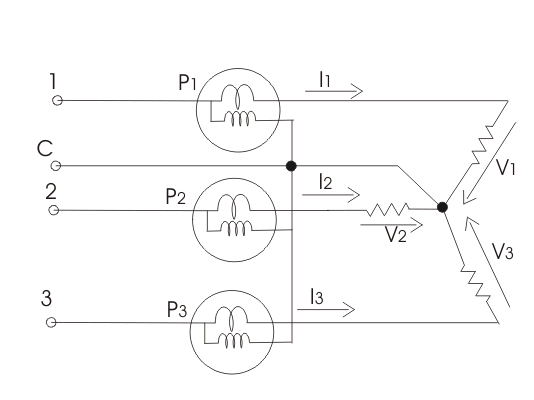Here, it is applied to three phase four wire systems, current coil of all the three wattmeters marked as 1, 2 and 3 are connected to respective phases marked as 1, 2 and 3. Pressure coils of all the three wattmeters are connected to a common point at neutral line. Clearly each wattmeter will give reading as product of phase current and line voltage which is phase power. The resultant sum of all the readings of wattmeter will give the total power of the circuit. Mathematically we can write## Measurement of Three Phase Power by Two Wattmeters Method

In this method we have two types of connections

When the load is star connected load, the diagram is shown in below-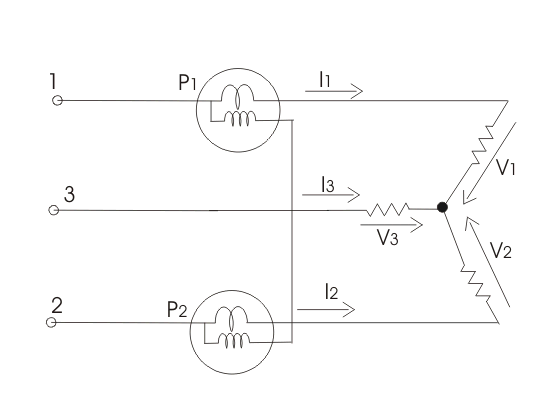For star connected load clearly the reading of wattmeter one is product of phase current and voltage difference (V2-V3). Similarly the reading of wattmeter two is the product of phase current and the voltage difference (V2-V3). Thus the total power of the circuit is sum of the reading of both the wattmeters. Mathematically we can write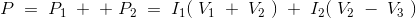but we have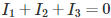, hence putting the value of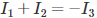.

We get total power as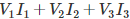.
When delta connected load, the diagram is shown in below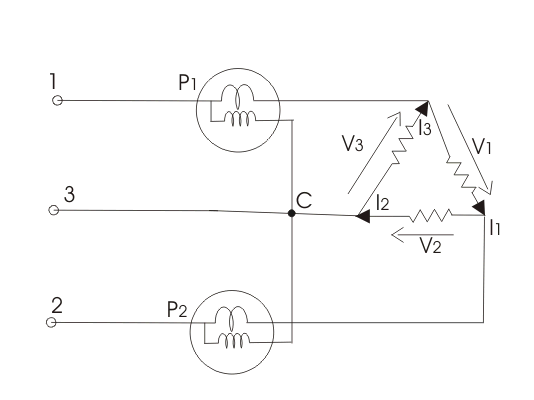The reading of wattmeter one can be written asand reading of wattmeter two is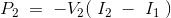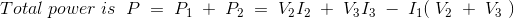but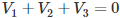, hence expression for total power will reduce to.

## Measurement of Three Phase Power by One Wattmeter Method

Limitation of this method is that it cannot be applied on unbalanced load. So under this condition we have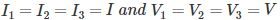.
Diagram is shown below: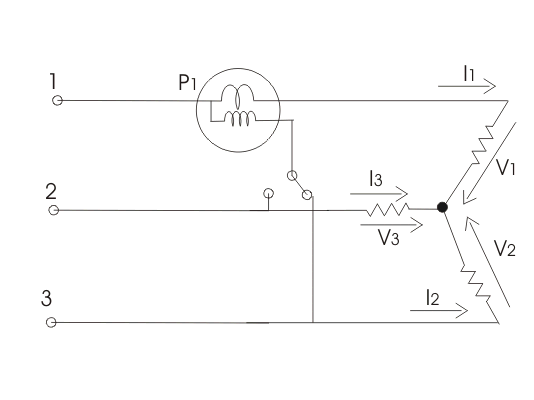Two switches are given which are marked as 1-3 and 1-2, by closing the switch 1-3 we get reading of wattmeter as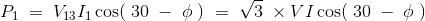Similarly the reading of wattmeter when switch 1-2 is closed is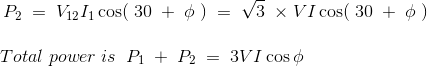Want To Learn Faster? 🎓
Get electrical articles delivered to your inbox every week.
No credit card required—it’s 100% free.